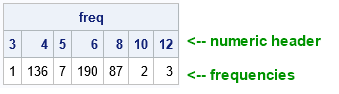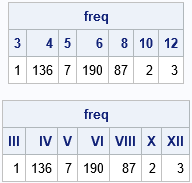Sometimes a little thing can make a big difference. I am enjoying a new enhancement of SAS/IML 15.1, which enables you to use a numeric vector as the column header or row header when you print a SAS/IML matrix. Prior to SAS/IML 15.1, you had to first use the CHAR or PUTN function to manually apply a format to the numeric values. You could then use the formatted values as column or row headers. Now you can skip the formatting step.

A common situation in which I want to use numeric values as a column header is when I am using the TABULATE function to compute the frequencies for a discrete numerical variable. For example, the Cylinders variable in the Sashelp.Cars data set indicates the number of cylinders in each vehicle. You might want to know how many vehicles in the data are four-cylinder, six-cylinder, eight-cylinder, and so forth. In SAS/IML 15.1, you can use a numeric variable (such as the levels of the Cylinders variable) directly in the COLNAME= option of the PRINT statement, as follows:

```proc iml; use Sashelp.Cars; read all var "Cylinders" into X; close;   call tabulate(level, freq, X); /* count number of obs for each level of X */ /* New in SAS/IML 15.1: use numeric values as COLNAME= option to PRINT statement */ print freq[colname=level];```Prior to SAS/IML 15.1, you had to convert numbers to character values by applying a format. This is commonly done by using the CHAR function, which applies a W.D format. This technique is still useful, but optional. You can also use the PUTN function to apply any format you want, such as COMMA., DATE., TIME., and so forth. For example, if you like Roman numerals, you could apply the ROMAN. format!

```labels = char(level, 2); /* apply w.d format */ print freq[colname=labels];   labels = putn(level, "ROMAN."); /* second arg is name of format */ print freq[colname=labels];```You can also use numeric values for the COLNAME= option to obtain row headers in SAS/IML 15.1. Sure, it's a small enhancement, but I like it!

The post Use numeric values for column headers when printing a matrix appeared first on The DO Loop.